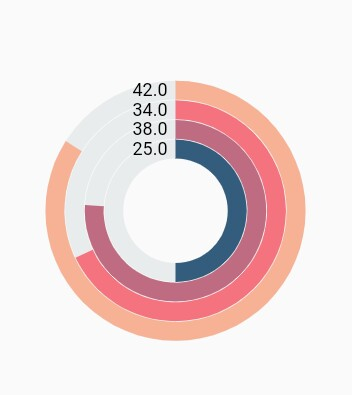# Chart types in Circular charts

8 Apr 2021 / 24 minutes to read

## Pie chart

To render a pie chart, create an instance of `PieSeries`, and add it to the `series` collection property of `SfCircularChart`. The following properties can be used to customize the appearance of pie segment:

• dart
• ``````@override
Widget build(BuildContext context) {
final List<ChartData> chartData = [
ChartData('David', 25),
ChartData('Steve', 38),
ChartData('Jack', 34),
ChartData('Others', 52)
];
return Scaffold(
body: Center(
child: Container(
child: SfCircularChart(
series: <CircularSeries>[
// Render pie chart
PieSeries<ChartData, String>(
dataSource: chartData,
pointColorMapper:(ChartData data,  _) => data.color,
xValueMapper: (ChartData data, _) => data.x,
yValueMapper: (ChartData data, _) => data.y
)
]
)
)
)
);
}

class ChartData {
ChartData(this.x, this.y, [this.color]);
final String x;
final double y;
final Color color;
}``````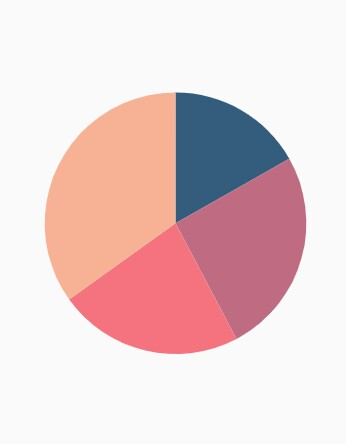### Changing pie size

You can use the `radius` property to change the diameter of the pie chart with respect to the plot area. The default value is 80%.

• dart
• ``````@override
Widget build(BuildContext context) {
return Scaffold(
body: Center(
child: Container(
child: SfCircularChart(
series: <CircularSeries>[
PieSeries<ChartData, String>(
dataSource: chartData,
xValueMapper: (ChartData data, _) => data.x,
yValueMapper: (ChartData data, _) => data.y,
// Radius of pie
radius: '50%'
)
]
)
)
)
);
}``````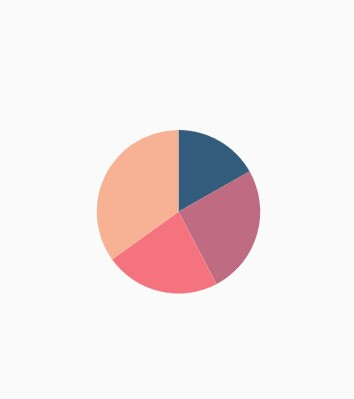### Exploding a segment

You can explode a pie segment by enabling the `explode` property. The following properties can be used to customize the explode options:

• dart
• ``````@override
Widget build(BuildContext context) {
return Scaffold(
body: Center(
child: Container(
child: SfCircularChart(
series: <CircularSeries>[
PieSeries<ChartData, String>(
dataSource: chartData,
xValueMapper: (ChartData data, _) => data.x,
yValueMapper: (ChartData data, _) => data.y,
// Segments will explode on tap
explode: true,
// First segment will be exploded on initial rendering
explodeIndex: 1
)
]
)
)
)
);
}``````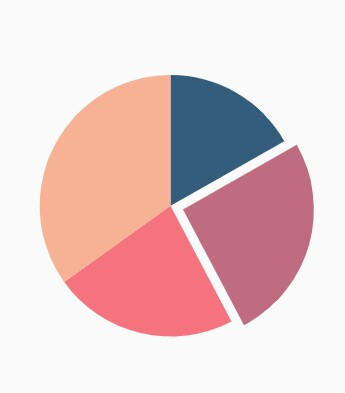### Exploding all the segments

Using the `explodeAll` property of `PieSeries`, you can explode all the pie segments.

• dart
• ``````@override
Widget build(BuildContext context) {
return Scaffold(
body: Center(
child: Container(
child: SfCircularChart(
series: <CircularSeries>[
PieSeries<ChartData, String>(
dataSource: chartData,
xValueMapper: (ChartData data, _) => data.x,
yValueMapper: (ChartData data, _) => data.y,
explode: true,
// All the segments will be exploded
explodeAll: true
)
]
)
)
)
);
}``````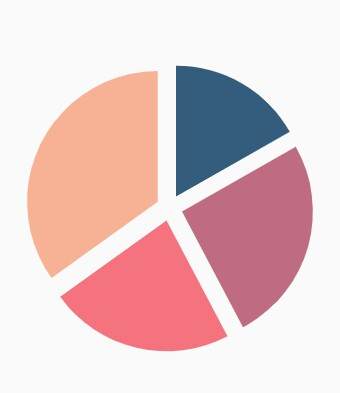### Angle of pie

`SfCircularChart` allows you to render all the data points or segments in semi-pie, quarter-pie, or in any sector using the `startAngle` and `endAngle` properties.

• dart
• ``````@override
Widget build(BuildContext context) {
return Scaffold(
body: Center(
child: Container(
child: SfCircularChart(
series: <CircularSeries>[
PieSeries<ChartData, String>(
dataSource: chartData,
xValueMapper: (ChartData data, _) => data.x,
yValueMapper: (ChartData data, _) => data.y,
startAngle: 270, // starting angle of pie
endAngle: 90 // ending angle of pie
)
]
)
)
)
);
}``````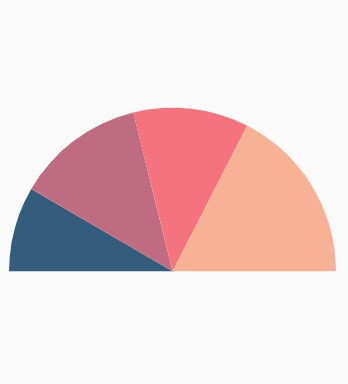### Grouping data points

The small segments in the pie chart can be grouped into others category using the `groupTo` and `groupMode` properties of `PieSeries`. The `groupMode` property specifies the grouping type based on the actual data point value or by points length, and the `groupTo` property sets the limit to group data points into a single slice. The grouped segment is labeled as Others in legend and toggled as any other segment. The default value of the `groupTo` property is null, and the default value of `groupMode` property is `point`.

• dart
• ``````@override
Widget build(BuildContext context) {
return Scaffold(
body: Center(
child: Container(
child: SfCircularChart(
series: <CircularSeries>[
PieSeries<ChartData, String>(
dataSource: chartData,
xValueMapper: (ChartData data, _) => data.x,
yValueMapper: (ChartData data, _) => data.y,
groupMode: CircularChartGroupMode.point,
// As the grouping mode is point, 2 points will be grouped
groupTo: 2
)
]
)
)
)
);
}``````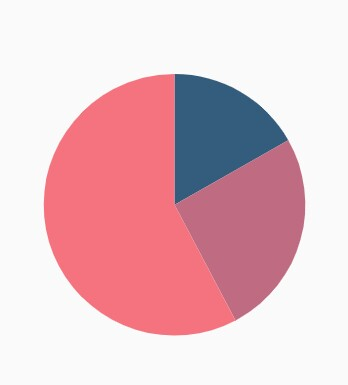### Various radius for each slice

The `pointRadiusMapper` maps the field name, which will be considered for calculating the radius of the data points.

• dart
• ``````@override
Widget build(BuildContext context) {
final List<ChartData> chartData = [
ChartData('USA', 10, '70%'),
ChartData('China', 11, '60%'),
ChartData('Russia', 9, '52%'),
ChartData('Germany', 10, '40%')
];
return Scaffold(
body: Center(
child: Container(
child: SfCircularChart(
series: <CircularSeries>[
PieSeries<ChartData, String>(
dataSource: chartData,
xValueMapper: (ChartData data, _) => data.x,
yValueMapper: (ChartData data, _) => data.y,
// Radius for each segment from data source
pointRadiusMapper: (ChartData data, _) => data.size
)
]
)
)
)
);
}

class ChartData {
ChartData(this.x, this.y, this.size);
final String x;
final double y;
final String size;
}``````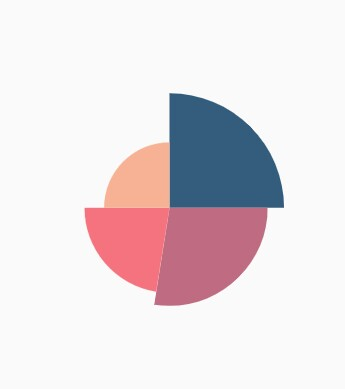## Doughnut chart

To render a doughnut chart, create an instance of `DoughnutSeries`, and add it to the `series` collection property of `SfCircularChart`. The following properties can be used to customize the appearance of doughnut segment:

• dart
• ``````@override
Widget build(BuildContext context) {
final List<ChartData> chartData = [
ChartData('David', 25, Color.fromRGBO(9,0,136,1)),
ChartData('Steve', 38, Color.fromRGBO(147,0,119,1)),
ChartData('Jack', 34, Color.fromRGBO(228,0,124,1)),
ChartData('Others', 52, Color.fromRGBO(255,189,57,1))
];
return Scaffold(
body: Center(
child: Container(
child: SfCircularChart(
series: <CircularSeries>[
// Renders doughnut chart
DoughnutSeries<ChartData, String>(
dataSource: chartData,
pointColorMapper:(ChartData data,  _) => data.color,
xValueMapper: (ChartData data, _) => data.x,
yValueMapper: (ChartData data, _) => data.y
)
]
)
)
)
);
}

class ChartData {
ChartData(this.x, this.y, [this.color]);
final String x;
final double y;
final Color color;
}``````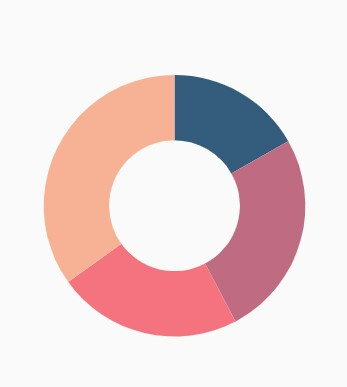### Rounded corners

The `cornerStyle` property specifies the corner type for doughnut chart. The corners can be customized using the `bothFlat`, `bothCurve`, `startCurve`, and `endCurve` options. The default value of this property is `bothFlat`.

• dart
• ``````@override
Widget build(BuildContext context) {
return Scaffold(
body: Center(
child: Container(
child: SfCircularChart(
series: <CircularSeries>[
DoughnutSeries<ChartData, String>(
dataSource: chartData,
xValueMapper: (ChartData data, _) => data.x,
yValueMapper: (ChartData data, _) => data.y,
// Corner style of doughnut segment
cornerStyle: CornerStyle.bothCurve
)
]
)
)
)
);
}``````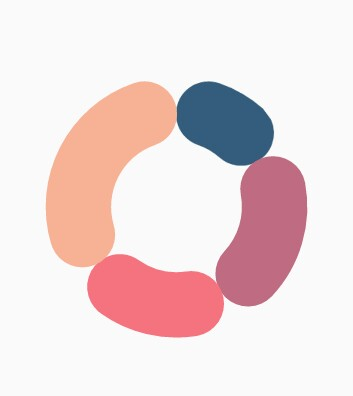### Doughnut with center elevation

You can use the Annotations property in charts, to provide center elevation text in doughnut charts as shown below:

• dart
• ``````@override
Widget build(BuildContext context) {
return Scaffold(
body: Center(
child: Container(
child: SfCircularChart(
annotations: <CircularChartAnnotation>[
CircularChartAnnotation(
child: Container(
child: PhysicalModel(
child: Container(),
shape: BoxShape.circle,
elevation: 10,
shadowColor: Colors.black,
color: const Color.fromRGBO(230, 230, 230, 1)))),
CircularChartAnnotation(
child: Container(
child: const Text('62%',
style: TextStyle(
color: Color.fromRGBO(0, 0, 0, 0.5), fontSize: 25))))
],
series: <CircularSeries>[
DoughnutSeries<ChartData, String>(
dataSource: chartData,
xValueMapper: (ChartData data, _) => data.x,
yValueMapper: (ChartData data, _) => data.y,
// Radius of doughnut
radius: '50%'
)
]
)
)
)
);
}``````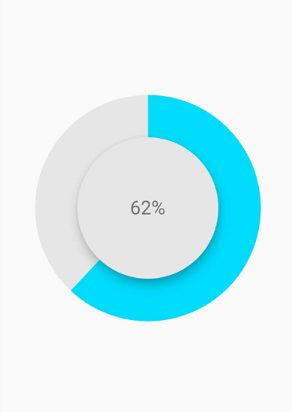### Doughnut with color mapping

You can use the `pointColorMapper` property to provide different color mappings to the doughnut charts as shown below:

• dart
• ``````@override
Widget build(BuildContext context) {
return Scaffold(
body: Center(
child: Container(
child: SfCircularChart(
series: <CircularSeries>[
DoughnutSeries<ChartData, String>(
dataSource: chartData,
xValueMapper: (ChartData data, _) => data.x,
yValueMapper: (ChartData data, _) => data.y,
pointColorMapper: (ChartSampleData data, _) => data.pointColor,
// Radius of doughnut
radius: '50%'
)
]
)
)
)
);
}``````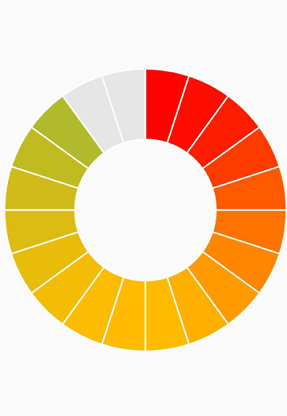### Changing the doughnut size

You can use the `radius` property to change the diameter of the doughnut chart with respect to the plot area. The default value of this property is 80%.

• dart
• ``````@override
Widget build(BuildContext context) {
return Scaffold(
body: Center(
child: Container(
child: SfCircularChart(
series: <CircularSeries>[
DoughnutSeries<ChartData, String>(
dataSource: chartData,
xValueMapper: (ChartData data, _) => data.x,
yValueMapper: (ChartData data, _) => data.y,
// Radius of doughnut
radius: '50%'
)
]
)
)
)
);
}``````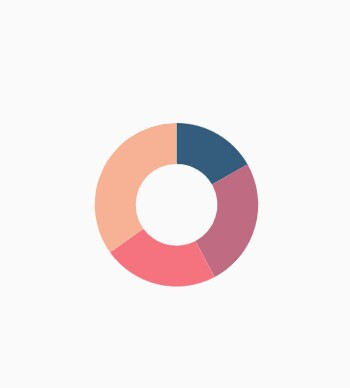### Changing doughnut inner radius

You can change the inner radius of doughnut chart using the `innerRadius` property with respect to the plot area. The value ranges from 0% to 100%.

• dart
• ``````@override
Widget build(BuildContext context) {
return Scaffold(
body: Center(
child: Container(
child: SfCircularChart(
series: <CircularSeries>[
DoughnutSeries<ChartData, String>(
dataSource: chartData,
xValueMapper: (ChartData data, _) => data.x,
yValueMapper: (ChartData data, _) => data.y,
// Radius of doughnut's inner circle
innerRadius: '80%'
)
]
)
)
)
);
}``````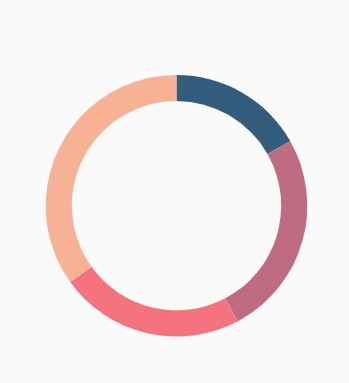### Exploding a segment

You can explode a doughnut segment by enabling the `explode` property. The following properties are used to customize the explode options:

• dart
• ``````@override
Widget build(BuildContext context) {
return Scaffold(
body: Center(
child: Container(
child: SfCircularChart(
series: <CircularSeries>[
DoughnutSeries<ChartData, String>(
dataSource: chartData,
xValueMapper: (ChartData data, _) => data.x,
yValueMapper: (ChartData data, _) => data.y,
// Explode the segments on tap
explode: true,
explodeIndex: 1
)
]
)
)
)
);
}``````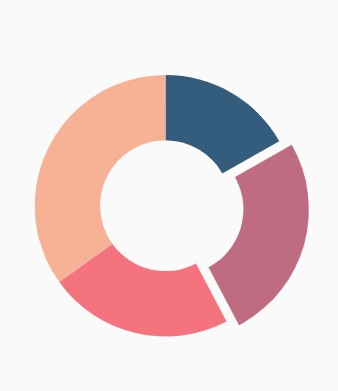### Exploding all the segments

Using the `explodeAll` property of `DoughnutSeries`, you can explode all the doughnut segments.

• dart
• ``````@override
Widget build(BuildContext context) {
return Scaffold(
body: Center(
child: Container(
child: SfCircularChart(
series: <CircularSeries>[
DoughnutSeries<ChartData, String>(
dataSource: chartData,
xValueMapper: (ChartData data, _) => data.x,
yValueMapper: (ChartData data, _) => data.y,
explode: true,
// Explode all the segments
explodeAll: true
)
]
)
)
)
);
}``````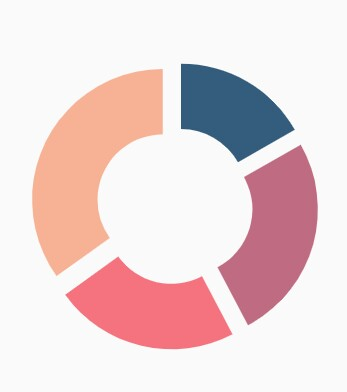### Angle of doughnut

`SfCircularChart` allows you to render all the data points or segments in semi-pie, quarter-pie, or in any sector using the `startAngle` and `endAngle` properties.

• dart
• ``````@override
Widget build(BuildContext context) {
return Scaffold(
body: Center(
child: Container(
child: SfCircularChart(
series: <CircularSeries>[
DoughnutSeries<ChartData, String>(
dataSource: chartData,
xValueMapper: (ChartData data, _) => data.x,
yValueMapper: (ChartData data, _) => data.y,
startAngle: 270, // Starting angle of doughnut
endAngle: 90 // Ending angle of doughnut
)
]
)
)
)
);
}``````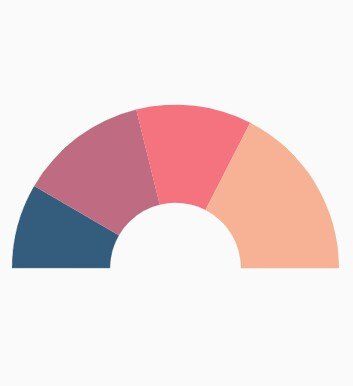### Grouping data points

The small segments in the doughnut chart can be grouped into others category using the `groupTo` and `groupMode` properties of `DoughnutSeries`. The `groupMode` property is used to specify the grouping type based on the actual data point value or by points length, and the `groupTo` property is used to set the limit to group data points into a single slice. The grouped segment is labeled as Others in legend and toggled as any other segment. The default value of the `groupTo` property is null, and the default value of `groupMode` property is `point`.

• dart
• ``````@override
Widget build(BuildContext context) {
return Scaffold(
body: Center(
child: Container(
child: SfCircularChart(
series: <CircularSeries>[
DoughnutSeries<ChartData, String>(
dataSource: chartData,
xValueMapper: (ChartData data, _) => data.x,
yValueMapper: (ChartData data, _) => data.y,
// Mode of grouping
groupMode: CircularChartGroupMode.point,
groupTo: 2
)
]
)
)
)
);
}``````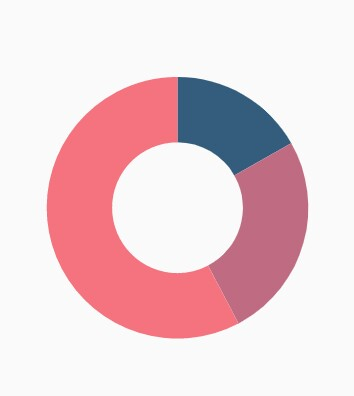## Radial bar chart

The radial bar chart is used for showing the comparisons among the categories using the circular shapes. To render a radial bar chart, create an instance of `RadialBarSeries`, and add to the `series` collection property of `SfCircularChart`. The following properties can be used to customize the appearance of radial bar segment:

• dart
• ``````@override
Widget build(BuildContext context) {
return Scaffold(
body: Center(
child: Container(
child: SfCircularChart(
series: <CircularSeries>[
// Renders radial bar chart
RadialBarSeries<ChartData, String>(
dataSource: chartData,
xValueMapper: (ChartData data, _) => data.x,
yValueMapper: (ChartData data, _) => data.y
)
]
)
)
)
);
}``````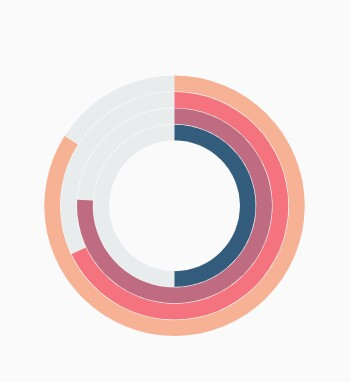### Changing the radial bar size

You can use the `radius` property to change the diameter of the radial bar chart with respect to the plot area. The default value is 80%.

• dart
• ``````@override
Widget build(BuildContext context) {
return Scaffold(
body: Center(
child: Container(
child: SfCircularChart(
series: <CircularSeries>[
RadialBarSeries<ChartData, String>(
dataSource: chartData,
xValueMapper: (ChartData data, _) => data.x,
yValueMapper: (ChartData data, _) => data.y,
// Radius of the radial bar
radius: '50%'
)
]
)
)
)
);
}``````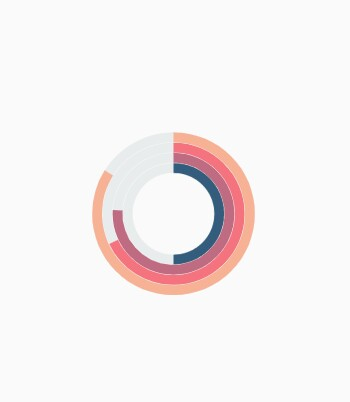### Changing the radial bar inner radius

You can change the inner radius of radial bar chart using the `innerRadius` property with respect to the plot area. The value ranges from 0% to 100%.

• dart
• ``````@override
Widget build(BuildContext context) {
return Scaffold(
body: Center(
child: Container(
child: SfCircularChart(
series: <CircularSeries>[
RadialBarSeries<ChartData, String>(
dataSource: chartData,
xValueMapper: (ChartData data, _) => data.x,
yValueMapper: (ChartData data, _) => data.y,
// Radius of the radial bar's inner circle
innerRadius: '80%'
)
]
)
)
)
);
}``````

### Rounded corners

The `cornerStyle` property specifies the corner type for radial bar chart. The corners can be customized using the `bothFlat`, `bothCurve`, `startCurve`, and `endCurve` options. The default value of this property is `bothFlat`.

• dart
• ``````@override
Widget build(BuildContext context) {
return Scaffold(
body: Center(
child: Container(
child: SfCircularChart(
series: <CircularSeries>[
RadialBarSeries<ChartData, String>(
dataSource: chartData,
xValueMapper: (ChartData data, _) => data.x,
yValueMapper: (ChartData data, _) => data.y,
// Corner style of radial bar segment
cornerStyle: CornerStyle.bothCurve
)
]
)
)
)
);
}``````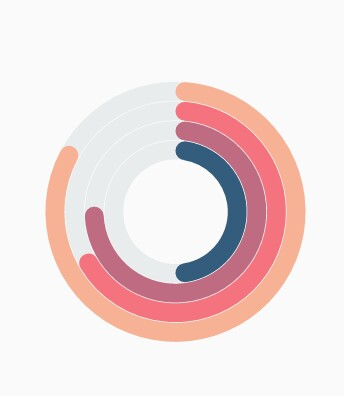### Rendering data labels

Data labels can be enabled using the `isVisible` property of `dataLabelSettings`. The appearance of label can be customized using the following properties:

• dart
• ``````@override
Widget build(BuildContext context) {
return Scaffold(
body: Center(
child: Container(
child: SfCircularChart(
series: <CircularSeries>[
RadialBarSeries<ChartData, String>(
dataSource: chartData,
xValueMapper: (ChartData data, _) => data.x,
yValueMapper: (ChartData data, _) => data.y,
dataLabelSettings: DataLabelSettings(
// Renders the data label
isVisible: true
)
)
]
)
)
)
);
}``````